Author: Oscar Cronquist Article last updated on May 11, 2022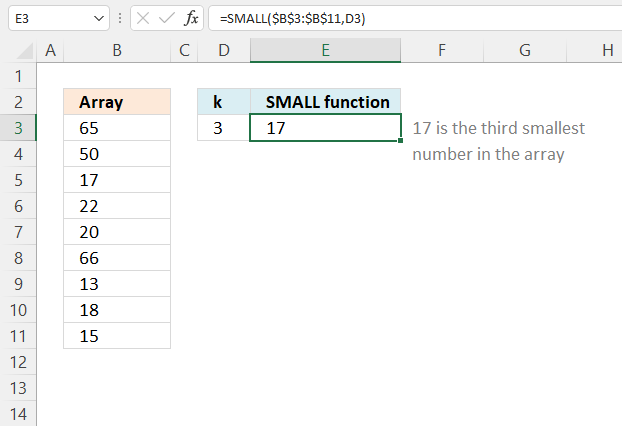The SMALL function returns the k-th smallest value from a group of numbers. The first argument is a cell range or array that you want to find the k-th smallest number in.

The second and last argument is k which is a number from 1 up to the number of values you have in the first argument.

Example shown in the above image, formula in cell E3 returns 17 because it is the third smallest number in cell range B3:B11.

=SMALL(B3:B11, D3)

Cell range B3:B1 contains the following numbers: 65, 50, 17, 22, 20, 66, 13, 18, and 15. Cell D3 contains the number that specifies which k-th smallest number to extract.

SMALL(array, k)

## 2. SMALL Function Arguments

 array Required. A group of numbers you want to extract the k-th smallest number from. k Required. k-th value, 1 returns the smallest number, 2 returns the second smallest number etc.

The SMALL function is very versatile and is, in my opinion, one of the most used functions in Microsoft Excel. You can construct both regular and array formulas with the SMALL function.

It also ignores blank values and text values, however, not error values. This article explains a workaround if you have error values in your data set.
How to ignore error values using the SMALL function

You can use a cell range across multiple columns like:

=SMALL(B3:D14, 2)

It will also work with multiple non-adjacent cell ranges with minor changes to the formula.

## 3. How does the SMALL function handle text and blank values?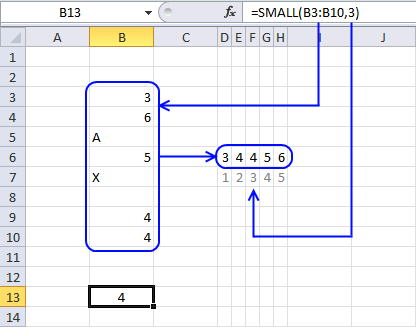The image above shows a formula in cell B13 that extracts the third smallest value from cell range B3:B10. Note that the cell range contains both text values and blank cells.

=SMALL(B3:B10, 3)

becomes

SMALL({3; 6; "A"; 5; "X"; 0; 4; 4}, 3)

Text strings and blanks are overlooked. The array becomes

SMALL({3; 6; ; 5; ; ; 4; 4}, 3)

and returns 4. 4 is the third smallest numerical value in the array.

## 4. How to use constants (hardcoded) values in the SMALL function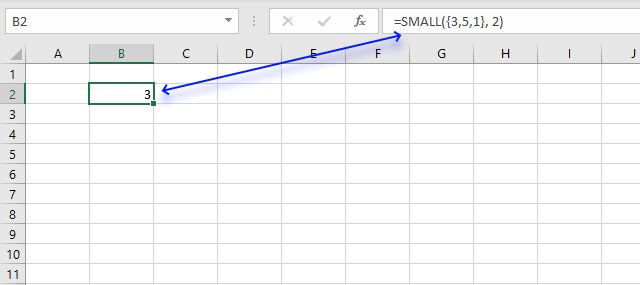In case you want to work with an array instead of a cell range in the SMALL function use curly brackets like this:

=SMALL({3, 5, 1}, 2)

This means that the values are hardcoded into the formula, however, you still enter it as a regular formula.

There is one downside with this approach and that is that you must edit the formula to be able to change a value in the array.

To convert a cell range to an array select the cell reference in the formula and press function key F9.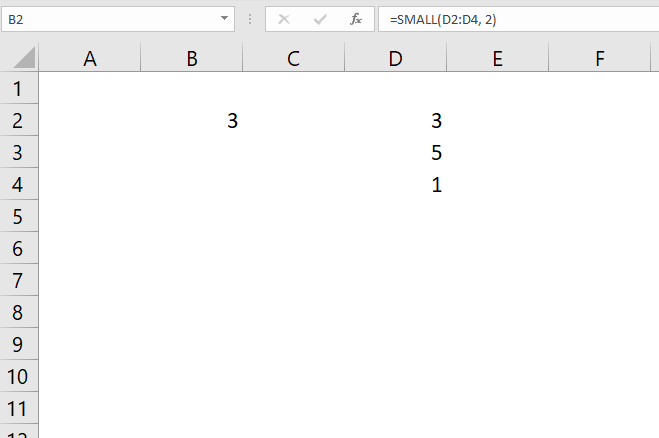This will convert the cell range to an array of values.

## 5. How to use a condition in the SMALL function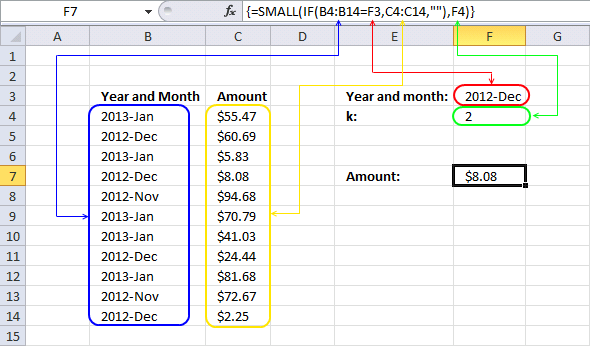This array formula in cell F7 calculates the second smallest number from cell range C4:C14 based on a condition specified in cell F3.

=SMALL(IF(B4:B14=F3, C4:C14, ""), F4)

The IF function returns one value if the logical test returns TRUE and another value if the logical test is FALSE.

IF(logical_test, [value_if_true], [value_if_false])

In this case, the IF function compares the values in cell range B4:B14 to the value in cell F3 and returns and an array that contains boolean values  TRUE or FALSE.

SMALL(IF(B4:B14=F3, C4:C14, ""), F4)

becomes

SMALL(IF({"2013-Jan"; "2012-Dec"; "2013-Jan"; "2012-Dec"; "2012-Nov"; "2013-Jan"; "2013-Jan"; "2012-Dec"; "2013-Jan"; "2012-Nov"; "2012-Dec"}="2012-Dec", C4:C14, ""), F4)

becomes

SMALL(IF({FALSE; TRUE; FALSE; TRUE; FALSE; FALSE; FALSE; TRUE; FALSE; FALSE; TRUE}, C4:C14, ""), F4)

The IF function then returns the corresponding value from the second argument if TRUE and the third argument if FALSE.

SMALL(IF({FALSE; TRUE; FALSE; TRUE; FALSE; FALSE; FALSE; TRUE; FALSE; FALSE; TRUE}, C4:C14, ""), F4)

becomes

SMALL(IF({FALSE; TRUE; FALSE; TRUE; FALSE; FALSE; FALSE; TRUE; FALSE; FALSE; TRUE},{55.47; 60.69; 5.83; 8.08; 94.68; 70.79; 41.03; 24.44; 81.68; 72.67; 2.25},""), F4)

becomes

SMALL({"";60.69;"";8.08;"";"";"";24.44;"";"";2.25}, 2)

and returns 8.08 in cell F7.

## 6. How to quickly return sorted numbers using the SMALL function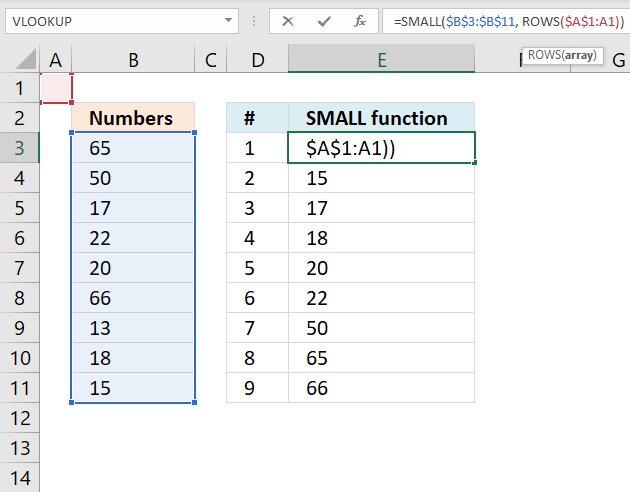Formula in cell E3:

=SMALL(\$B\$3:\$B\$11, ROWS(\$A\$1:A1))

The second argument k can be changed from a number to a function that returns numbers, this can be handy when you want to return multiple numbers sorted from small to large.

SMALL(array, k )

The ROWS function returns the number of rows a cell range contains. If you combine absolute and relative references into one cell reference you can build a dynamic cell reference that changes when you copy the cell and paste to cells below.

\$A\$1:A1

The first part of the cell reference is absolute meaning it won't change when the cell is copied and pasted to cells below. You can see that it is absolute bu the \$ dollar signs in front of the column letter and the row number.

The colon is used to describe a cell range that contains multiple cells however it can also describe a reference to a single cell. The second part is relative meaning it will change when you copy the cell.

For example, the table below demonstrates how the cell references in the formula change when copied.

Cell E3: =SMALL(\$B\$3:\$B\$11, ROWS(\$A\$1:A1))

Cell E4: =SMALL(\$B\$3:\$B\$11, ROWS(\$A\$1:A2))

Cell E5: =SMALL(\$B\$3:\$B\$11, ROWS(\$A\$1:A3))

Note that you need to copy the cell not the formula to take advantage of growing cell references.

The cell range expands by one row for each new cell below you paste it to. The ROWS function calculates the number of rows in that cell range and returns that number.

Cell E3: =SMALL(\$B\$3:\$B\$11, 1)

Cell E4: =SMALL(\$B\$3:\$B\$11, 2)

Cell E5: =SMALL(\$B\$3:\$B\$11, 3)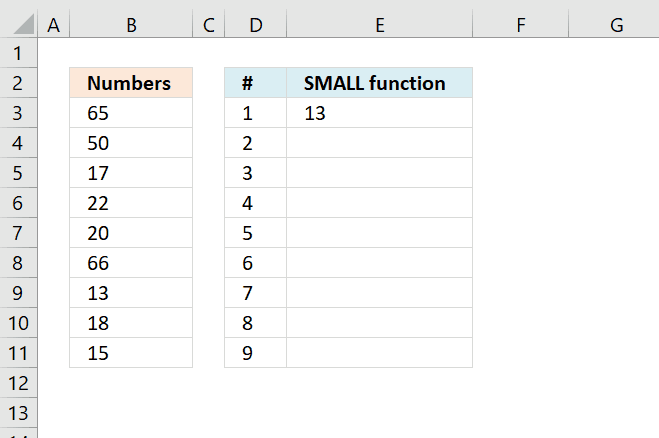You can press and hold on the black dot in the bottom right corner of the selected cell then drag down as far as needed to quickly copy the cell to cells below, see animated image above.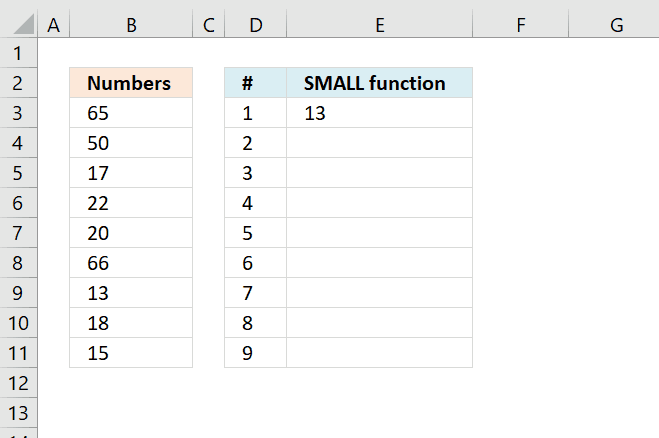You can also double press with left mouse button on with left mouse button on the black dot located in the bottom right corner of the selected cell to quickly copy the cell to cells below.

Excel uses existing values in the adjacent column to determine when to stop copying.